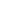## Structure & Bonding

Molecules of organic compounds are made up of discrete collections of atoms that are held together (bonded) in three-dimensional space in a unique constitution and configuration, referred to as its structure. The study of organic chemistry must therefore extend to the molecular level, for the physical and chemical properties of a substance are ultimately explained in terms of the structure and bonding of molecules. This chapter introduces some basic facts and principles that are needed for a discussion of organic molecules.

### Electron Configurations in the Periodic Table

 1A 2A 3A 4A 5A 6A 7A 8A 1H1s1 2He1s2 3Li1s22s1 4Be1s22s2 5B1s22s22p1 6C1s22s22p2 7N1s22s22p3 8O1s22s22p4 9F1s22s22p5 10Ne1s22s22p6 11Na[Ne]3s1 12Mg[Ne]3s2 13Al[Ne]3s23p1 14Si[Ne]3s23p2 15P[Ne]3s23p3 16S[Ne]3s23p4 17Cl[Ne]3s23p5 18Ar[Ne]3s23p6
The periodic table shown here is severely truncated; there are over eighty other elements.

Four elements, hydrogen, carbon, oxygen and nitrogen, are the major components of most organic compounds. Consequently, our understanding of organic chemistry must have, as a foundation, an appreciation of the electronic structure and properties of these elements. The truncated periodic table shown above provides the orbital electronic structure for the first eighteen elements (hydrogen through argon). According to the Aufbau principle, the electrons of an atom occupy quantum levels or orbitals starting from the lowest energy level, and proceeding to the highest, with each orbital holding a maximum of two paired electrons (opposite spins).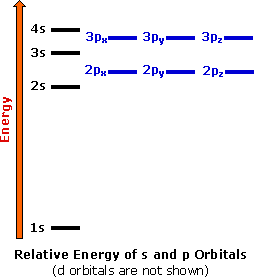Electron shell #1 has the lowest energy and its s-orbital is the first to be filled. Shell #2 has four higher energy orbitals, the 2s-orbital being lower in energy than the three 2p-orbitals. (x, y & z). As we progress from lithium (atomic number=3) to neon (atomic number=10) across the second row or period of the table, all these atoms start with a filled 1s-orbital, and the 2s-orbital is occupied with an electron pair before the 2p-orbitals are filled. In the third period of the table, the atoms all have a neon-like core of 10 electrons, and shell #3 is occupied progressively with eight electrons, starting with the 3s-orbital. The highest occupied electron shell is called the valence shell, and the electrons occupying this shell are called valence electrons.

The chemical properties of the elements reflect their electron configurations. For example, helium, neon and argon are exceptionally stable and unreactive monoatomic gases. Helium is unique since its valence shell consists of a single s-orbital. The other members of group 8 have a characteristic valence shell electron octet (ns2 + npx2 + npy2 + npz2). This group of inert (or noble) gases also includes krypton (Kr: 4s2, 4p6), xenon (Xe: 5s2, 5p6) and radon (Rn: 6s2, 6p6). In the periodic table above these elements are colored beige.
The halogens (F, Cl, Br etc.) are one electron short of a valence shell octet, and are among the most reactive of the elements (they are colored red in this periodic table). In their chemical reactions halogen atoms achieve a valence shell octet by capturing or borrowing the eighth electron from another atom or molecule. The alkali metals Li, Na, K etc. (colored violet above) are also exceptionally reactive, but for the opposite reason. These atoms have only one electron in the valence shell, and on losing this electron arrive at the lower shell valence octet. As a consequence of this electron loss, these elements are commonly encountered as cations (positively charged atoms).
The elements in groups 2 through 7 all exhibit characteristic reactivities and bonding patterns that can in large part be rationalized by their electron configurations. It should be noted that hydrogen is unique. Its location in the periodic table should not suggest a kinship to the chemistry of the alkali metals, and its role in the structure and properties of organic compounds is unlike that of any other element.

### Chemical Bonding and Valence

As noted earlier, the inert gas elements of group 8 exist as monoatomic gases, and do not in general react with other elements. In contrast, other gaseous elements exist as diatomic molecules (H2, N2, O2, F2 & Cl2), and all but nitrogen are quite reactive. Some dramatic examples of this reactivity are shown in the following equations.
 2 Na + Cl22 NaCl 2 H2 + O22 H2O C + O2CO2 C + 2 F2CF4

Why do the atoms of many elements interact with each other and with other elements to give stable molecules? In addressing this question it is instructive to begin with a very simple model for the attraction or bonding of atoms to each other, and then progress to more sophisticated explanations.

### 1. Ionic Bonding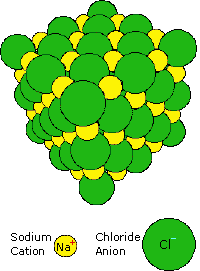When sodium is burned in a chlorine atmosphere, it produces the compound sodium chloride. This has a high melting point (800 ºC) and dissolves in water to to give a conducting solution. Sodium chloride is an ionic compound, and the crystalline solid has the structure shown on the right. Transfer of the lone 3s electron of a sodium atom to the half-filled 3p orbital of a chlorine atom generates a sodium cation (neon valence shell) and a chloride anion (argon valence shell), as shown in the following equation. Electrostatic attraction results in these oppositely charged ions packing together in a lattice (structure on the right). The attractive forces holding the ions in place can be referred to as ionic bonds.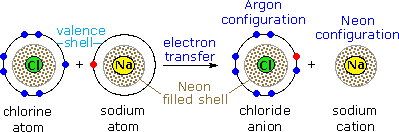By clicking on the NaCl diagram, a model of this crystal will be displayed and may be manipulated.

### 2. Covalent Bonding

The other three reactions shown above give products that are very different from sodium chloride. Water is a liquid at room temperature; carbon dioxide and carbon tetrafluoride are gases. None of these compounds is composed of ions. A different attractive interaction between atoms, called covalent bonding, is involved here. Covalent bonding occurs by a sharing of valence electrons, rather than an outright electron transfer. Similarities in physical properties (they are all gases) suggest that the diatomic elements H2, N2, O2, F2 & Cl2 also have covalent bonds.
Examples of covalent bonding shown below include hydrogen, fluorine, carbon dioxide and carbon tetrafluoride. These illustrations use a simple Bohr notation, with valence electrons designated by colored dots. Note that in the first case both hydrogen atoms achieve a helium-like pair of 1s-electrons by sharing. In the other examples carbon, oxygen and fluorine achieve neon-like valence octets by a similar sharing of electron pairs. Carbon dioxide is notable because it is a case in which two pairs of electrons (four in all) are shared by the same two atoms. This is an example of a double covalent bond.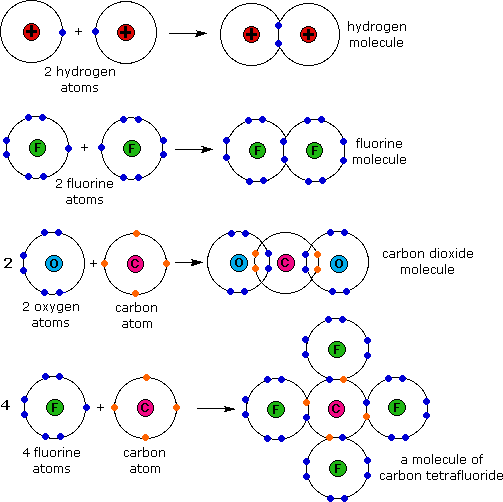These electron sharing diagrams (Lewis formulas) are a useful first step in understanding covalent bonding, but it is quicker and easier to draw Couper-Kekulé formulas in which each shared electron pair is represented by a line between the atom symbols. Non-bonding valence electrons are shown as dots. These formulas are derived from the graphic notations suggested in 1857 by A. Couper and A. Kekulé, and are not identical to their original drawings. Some examples of such structural formulas are given in the following table.

Common Name Molecular Formula Lewis Formula Kekulé Formula
Methane CH4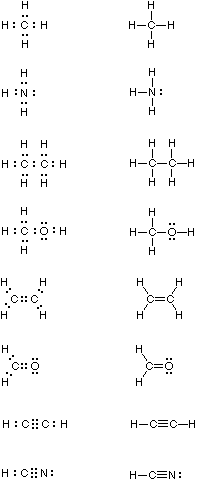Ammonia NH3
Ethane C2H6
Methyl Alcohol CH4O
Ethylene C2H4
Formaldehyde CH2O
Acetylene C2H2
Hydrogen Cyanide CHN

Multiple bonding, the sharing of two or more electron pairs, is illustrated by ethylene and formaldehyde (each has a double bond), and acetylene and hydrogen cyanide (each with a triple bond). Boron compounds such as BH3 and BF3 are exceptional in that conventional covalent bonding does not expand the valence shell occupancy of boron to an octet. Consequently, these compounds have an affinity for electrons, and they exhibit exceptional reactivity when compared with the compounds shown above.

### 3. Valence

The number of valence shell electrons an atom must gain or lose to achieve a valence octet is called valence. In covalent compounds the number of bonds which are characteristically formed by a given atom is equal to that atom's valence. From the formulas written above, we arrive at the following general valence assignments:

 Atom H C N O F Cl Br I Valence 1 4 3 2 1 1 1 1

The valences noted here represent the most common form these elements assume in organic compounds. Many elements, such as chlorine, bromine and iodine, are known to exist in several valence states in different inorganic compounds.

### The Shape of Molecules

The three dimensional shape or configuration of a molecule is an important characteristic. This shape is dependent on the preferred spatial orientation of covalent bonds to atoms having two or more bonding partners. Three dimensional configurations are best viewed with the aid of models.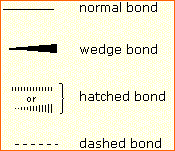In order to represent such configurations on a two-dimensional surface (paper, blackboard or screen), we often use perspective drawings in which the direction of a bond is specified by the line connecting the bonded atoms.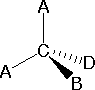In most cases the focus of configuration is a carbon atom so the lines specifying bond directions will originate there. As defined in the diagram on the right, a simple straight line represents a bond lying approximately in the surface plane. The two bonds to substituents A in the structure on the left are of this kind. A wedge shaped bond is directed in front of this plane (thick end toward the viewer), as shown by the bond to substituent B; and a hatched bond is directed in back of the plane (away from the viewer), as shown by the bond to substituent D. Some texts and other sources may use a dashed bond in the same manner as we have defined the hatched bond, but this can be confusing because the dashed bond is often used to represent a partial bond (i.e. a covalent bond that is partially formed or partially broken). The following examples make use of this notation, and also illustrate the importance of including non-bonding valence shell electron pairs (colored blue) when viewing such configurations .
If we consider only the shape created by the atoms themselves, these structures appear to be respectively tetrahedral, pyramidal and bent, shown in red by Clicking on the Diagram. When the non-bonding valence electrons are included in the structure, all of these examples have a tetrahedral configuration, shown by Clicking on the Diagram a second time.

Bonding configurations are readily predicted by valence-shell electron-pair repulsion theory, commonly referred to as VSEPR in most introductory chemistry texts. This simple model is based on the fact that electrons repel each other, and that it is reasonable to expect that the bonds and non-bonding valence electron pairs associated with a given atom will prefer to be as far apart as possible. The bonding configurations of carbon are easy to remember, since there are only three categories.

Configuration Bonding Partners Bond Angles Example
Tetrahedral 4 109.5º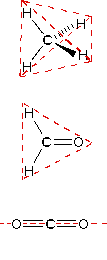Trigonal 3 120º
Linear 2 180º

In the three examples shown above, the central atom (carbon) does not have any non-bonding valence electrons; consequently the configuration may be estimated from the number of bonding partners alone. For molecules of water and ammonia, however, the non-bonding electrons must be included in the calculation. In each case there are four regions of electron density associated with the valence shell so that a tetrahedral bond angle is expected. The measured bond angles of these compounds (H2O 104.5º & NH3 107.3º) show that they are closer to being tetrahedral than trigonal or linear. The compound boron trifluoride, BF3, does not have non-bonding valence electrons and the configuration of its atoms is trigonal.

Nice treatments of VSEPR theory have been provided by Oxford and Purdue .
Click on the university name to visit their site.

The best way to study the three-dimensional shapes of molecules is by using molecular models. Many kinds of model kits are available to students and professional chemists. Some of the useful features of physical models can be approximated by the model viewing applet Jmol. This powerful visualization tool allows the user to move a molecular stucture in any way desired. Atom distances and angles are easily determined. To measure a distance, double-click on two atoms. To measure a bond angle, do a double-click, single-click, double-click on three atoms. To measure a torsion angle, do a double-click, single-click, single-click, double-click on four atoms. A pop-up menu of commands may be accessed by the right button on a PC or a control-click on a Mac while the cursor is inside the display frame.
You may examine several Jmol models of compounds discussed above by Clicking Here.

### Hypervalent Compounds

The concept of valence given above assumes the octet rule is always obeyed, which is generally the case for second row elements. When compounds of third row elements are examined, structures analogous to methane, ammonia and water are found, but higher valent compounds that appear to violate the octet rule also exist. Three examples are given in the following table. The penta and hexavalent compounds of silicon, phosphorous and sulfur adopt trigonal bipyramidal and octahedral molecular structures, as predicted by VSEPR. Clicking on the table will display examples of these shapes. The covalent bonding in such compounds involves participation of low energy 3d-orbitals,and will be discussed in a later section of this chapter.The hexafluorides, sulfur hexafluoride,SF6, and hexafluorosilicic acid, H2SiF6, both have octahedral structures. From a valence shell electron count. the former is neutral, with a partial positive charge on sulfur and balancing negative charges on fluorine. The latter is a moderately strong acid stable only in water solution. Its salts have seen use as a pesticide. The useful chlorinating agent phosphorous pentachloride, PCl5, is formed by reacting PCl3 with Cl2. It decomposes to these reactants on heating.

### Structural Formulas

It is necessary to draw structural formulas for organic compounds because in most cases a molecular formula does not uniquely represent a single compound. Different compounds having the same molecular formula are called isomers, and the prevalence of isomers among organic compounds reflects the extraordinary versatility of carbon in forming strong bonds to itself and to other elements.
When the group of atoms that make up the molecules of different isomers are bonded together in fundamentally different ways, we refer to such compounds as constitutional isomers. There are seven constitutional isomers of C4H10O, and structural formulas for these are drawn in the following table. These formulas represent all known and possible C4H10O compounds, and display a common structural feature. There are no double or triple bonds and no rings in any of these structures. Curiously, if two hydrogens are removed from the molecular formula, giving C4H8O, the number of constitutional isomers increases to eighteen, and all these compounds have either a ring or a double bond in their structural formulas. The reason for this will be discussed in the bext section.

#### Structural Formulas for C4H10O Isomers

Kekulé Formula Condensed Formula Shorthand Formula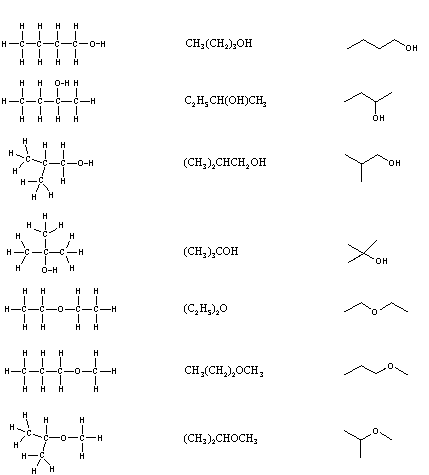Simplification of structural formulas may be achieved without any loss of the information they convey. In condensed structural formulas the bonds to each carbon are omitted, but each distinct structural unit (group) is written with subscript numbers designating multiple substituents, including the hydrogens. Shorthand (line) formulas omit the symbols for carbon and hydrogen entirely. Each straight line segment represents a bond, the ends and intersections of the lines are carbon atoms, and the correct number of hydrogens is calculated from the tetravalency of carbon. Non-bonding valence shell electrons are omitted in these formulas.
Developing the ability to visualize a three-dimensional structure from two-dimensional formulas requires practice, and in most cases the aid of molecular models. As noted earlier, many kinds of model kits are available to students and professional chemists, and the beginning student is encouraged to obtain one.
Although constitutional isomers have the same molecular formula, their physical and chemical properties may be very different. For an example Click Here.

### Analysis of Molecular Formulas

Even though structural formulas are essential to the unique description of organic compounds, it is interesting and instructive to evaluate the information that may be obtained from a molecular formula alone. Three useful rules may be listed:

1. The number of hydrogen atoms that can be bonded to a given number of carbon atoms is limited by the valence of carbon. For compounds of carbon and hydrogen (hydrocarbons) the maximum number of hydrogen atoms that can be bonded to n carbons is 2n + 2 (n is an integer). In the case of methane, CH4, n=1 & 2n + 2 = 4. The origin of this formula is evident by considering a hydrocarbon made up of a chain of carbon atoms. Here the middle carbons will each have two hydrogens and the two end carbons have three hydrogens each. Thus, a six-carbon chain (n = 6) may be written H-(CH2)6-H, and the total hydrogen count is (2 x 6) + 2 = 14. The presence of oxygen (valence = 2) does not change this relationship, so the previously described C4H10O isomers follow the rule, n=4 & 2n + 2 = 10. Halogen atoms (valence = 1) should be counted equivalent to hydrogen, as illustrated by C3H5Cl3, n = 3 & 2n + 2 = 8 = (5 + 3). If nitrogen is present, each nitrogen atom (valence = 3) increases the maximum number of hydrogens by one.
 Some PlausibleMolecular Formulas C7H16O3, C9H18, C15H28O3, C6H16N2 Some ImpossibleMolecular Formulas C8H20O6, C23H50, C5H10Cl4, C4H12NO
2. For stable organic compounds the total number of odd-valenced atoms is even. Thus, when even-valenced atoms such as carbon and oxygen are bonded together in any number and in any manner, the number of remaining unoccupied bonding sites must be even. If these sites are occupied by univalent atoms such as H, F, Cl, etc. their total number will necessarily be even. Nitrogen is also an odd-valenced atom (3), and if it occupies a bonding site on carbon it adds two additional bonding sites, thus maintaining the even/odd parity.
 Some PlausibleMolecular Formulas C4H4Cl2, C5H9OBr, C5H11NO2, C12H18N2FCl Some ImpossibleMolecular Formulas C5H9O2, C4H5ClBr, C6H11N2O, C10H18NCl2
3. The number of hydrogen atoms in stable compounds of carbon, hydrogen & oxygen reflects the number of double bonds and rings in their structural formulas. Consider a hydrocarbon with a molecular structure consisting of a simple chain of four carbon atoms, CH3CH2CH2CH3. The molecular formula is C4H10 (the maximum number of bonded hydrogens by the 2n + 2 rule). If the four carbon atoms form a ring, two hydrogens must be lost. Similarly, the introduction of a double bond entails the loss of two hydrogens, and a triple bond the loss of four hydrogens.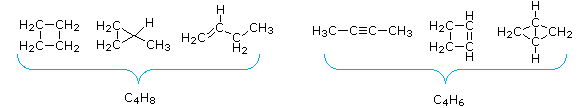From the above discussion and examples it should be clear that the molecular formula of a hydrocarbon (CnHm) provides information about the number of rings and/or double bonds that must be present in its structural formula. By rule #2 m must be an even number, so if m < (2n + 2) the difference is also an even number that reflects any rings and double bonds. A triple bond is counted as two double bonds.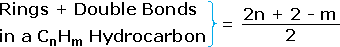The presence of one or more nitrogen atoms or halogen substituents requires a modified analysis. The above formula may be extended to such compounds by a few simple principles:

• The presence of oxygen does not alter the relationship.
• All halogens present in the molecular formula must be replaced by hydrogen.
• Each nitrogen in the formula must be replaced by a CH moiety.

### Structural Equivalence of Carbon Atoms

When discussing structural formulas, it is often useful to distinguish different groups of carbon atoms by their structural characteristics. A primary carbon (1º) is one that is bonded to no more than one other carbon atom. A secondary carbon (2º) is bonded to two other carbon atoms, and tertiary (3º) and quaternary (4º) carbon atoms are bonded respectively to three and four other carbons. The three C5H12 isomers shown below illustrate these terms.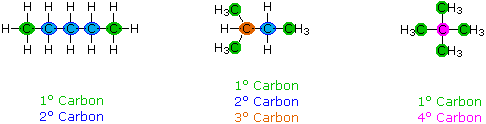Structural differences may occur within these four groups, depending on the molecular constitution. In the formula on the right all four 1º-carbons are structurally equivalent (remember the tetrahedral configuration of tetravalent carbon); however the central formula has two equivalent 1º-carbons (bonded to the 3º carbon on the left end) and a single, structurally different 1º-carbon (bonded to the 2º-carbon) at the right end. Similarly, the left-most formula has two structurally equivalent 2º-carbons (next to the ends of the chain), and a structurally different 2º-carbon in the middle of the chain. A consideration of molecular symmetry helps to distinguish structurally equivalent from nonequivalent atoms and groups. The ability to distinguish structural differences of this kind is an essential part of mastering organic chemistry. It will come with practice and experience.

Individual molecules are too small to be viewed by even powerful microscopes.
To see how chemists can draw structural formulas with confidence Click Here.

 Practice Problems

The following problems explore many of the concepts discussed above. They include valency, evaluation of line and condensed structural formulas, analyzing molecular formulas and identifying structurally equivalent groups.